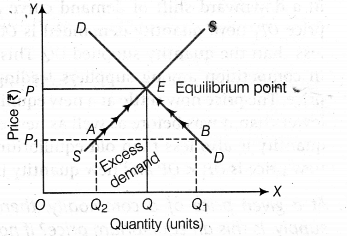# At a given price of a commodity, there is excess demand

At a given price of a commodity, there is excess demand. Is this price an equilibrium price? If not, how will the equilibrium price be reached?

By the definition, equilibrium price refers to the price at which market demand is equal to market supply (i.e. there is no excess demand or excess supply).
So, the price with excess demand is not an equilibrium price. This can be illustrated with the help of the given figure.In this figure, at {{OP}_{1}} level of price, AB is equal to
{{Q}_{1}}
{{Q}_{2}} i.e. excess demand. With the market demand greater than market supply, the pressure exists to move the price upwards. The quantity supplied tends to expands in correspondence with rising price because producers supply more at higher price and movement along the supply curve SS from point A to point E. At higher price OP, quantity supplied increases from
{{OQ}_{2}} to OQ. Consumers react to rising price by reducing consumptions. Hence, movement along demand curve DD from point B to point E. Thus, quantity demanded falls from
{{OQ}_{1}} to OQ.
The equilibrium 'is struck at point E with OP and OQ as the equilibrium price and equilibrium quantity respectively. At OP price, there is no excess demand.